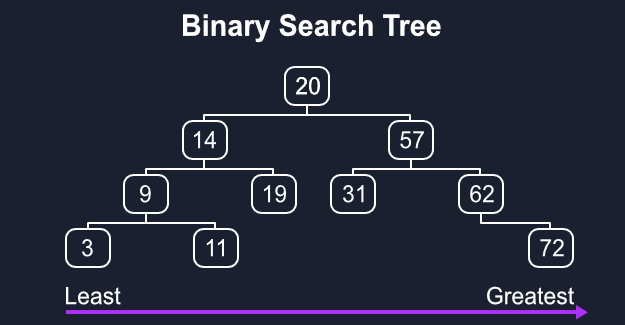# Binary Search Trees Through JavaScript

The problem with normal tree structures is that they are unsorted and very unwieldy to work with. If you run a search for a file on your computer it’ll generally take a pretty long time, this is because your files are unsorted and it has to search through EVERYTHING to get a result. We can heavily improve this by upgrading how we organize the values in our trees.

## Prerequisites

You’ll need to know the basic concepts of trees, which you can learn here, we’re also going to need to do some very basic tree traversal with a breadth-first search, which you can brush up on here.

## Concept

A binary tree is just a normal tree with the limitation of each node only being able to, at most, have two children. A binary search tree just has the additional rule that if there’s two values then they need to be ordered, in our case from the lower number on the left to the higher on the right.

Searching on a binary search tree is a large improvement on our original `O(n)` search speed since now to find something we just need to compare what we want to each parent node before moving left or right until we’re at what we want, giving us `O(logn)` for all operations.## Create

Very similar to linked lists we can use classes to generate our nodes and tree. Each node only really needs a pointer to the left/less and right/greater sides, the value, and I personally like to add a counter since repeated values can only exist once in the tree.

After checking if there’s anything already there, we can use a nice little utility function to put our value to a side. Very simply, we just need to loop through the tree, if our value is less than the current node move left else move right, if there’s nothing there become the new node in that position, if a matching value’s already there then we can increment its counter.

``````class Node {
constructor(val) {
this.val = val;
this.right = null;
this.left = null;
this.count = 0;
};
};

class BST {
constructor() {
this.root = null;
}
create(val) {
const newNode = new Node(val);
if (!this.root) {
this.root = newNode;
return this;
};
let current = this.root;

const addSide = side => {
if (!current[side]) {
current[side] = newNode;
return this;
};
current = current[side];
};

while (true) {
if (val === current.val) {
current.count++;
return this;
};
};
};
};

let tree = new BST();
console.log(tree);
``````

## Find

Finding something is incredibly simple, just move left or right relative to the current value and return `true` if we hit something that matches.

``````find(val) {
if (!this.root) return undefined;
let current = this.root,
found = false;

while (current && !found) {
if (val < current.val) current = current.left;
else if (val > current.val) current = current.right;
else found = true;
};

if (!found) return 'Nothing Found!';
return current;
};
``````

## Delete

Deletion are the most complicated operation since we’re not working with just leafs but having to restructure, or “rebalance”, all of a node’s children. There are 2 conditions we have to check for, whether the node is a leaf and if it has children.

First we need a utility function to collect all of the deleted node’s children. I’ll use a basic breadth-first search to push everything into an array which we can then loop through to re-add each item to the tree.

The only difference from a normal search is that it needs to accept a different starting point, so we can limit our search only to the subtree of our deleted node’s children.

``````BFS(start) {
let data = [],
queue = [],
current = start ? this.find(start) : this.root;

queue.push(current);
while (queue.length) {
current = queue.shift();
data.push(current.val);

if (current.left) queue.push(current.left);
if (current.right) queue.push(current.right);
};

return data;
}
``````

Since we can’t traverse back up to the parent we’ll use a variable to store the parent node to `current` and use that to set `current` to `null` after we’ve saved the children.

In `deleteNode` we’ll collect our node’s children, set it on its parent to `null`, then use `create` on each of the children, restructuring them properly in the tree. Our children array will also include the deleted node, which we can just splice out.

``````delete(val) {
if (!this.root) return undefined;
let current = this.root,
parent;

const pickSide = side => {
if (!current[side]) return 'No node found!';

parent = current;
current = current[side];
};

const deleteNode = side => {
if (current.val === val && current.count > 1) current.count--;
else if (current.val === val) {
const children = this.BFS(current.val);
parent[side] = null;
children.splice(0, 1);
children.forEach(child => this.create(child));
};
};

while (current.val !== val) {
if (val < current.val) {
pickSide('left');
deleteNode('left');
};
else {
pickSide('right');
deleteNode('right');
};
};

return current;
}
``````

## Conclusion

This would have been the full CRUD operations, as any update is essentially just deleting one node and creating a whole new one somewhere else, which doesn’t really need its own method.

We’ll be able to do some cooler stuff with binary search trees as we move into binary heaps and priority queues.

🔎 Searching...